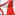PROGRAM SYSTEMS: THEORY AND APPLICATIONS 12+Papers are accepted in the form of a PDF file To view the PDF files, you will need Adobe Acrobat Reader• Содержание выпуска •
• Methods for Optimal Control and Control Theory •
• Software and Hardware for Distributed Systems and Supercomputers •
• Mathematical Foundations of Programming •
• Artificial Intelligence, Intelligence Systems, Neural Networks •
• Mathematical Modelling •

# Mathematical Foundations of Programming

Responsible for the Section: doctor of physico-mathematical Sciences Nikolay Nepeivoda

On the left: assigned number of the paper, submission date, the number of A5 pages contained in the paper, and the reference to the full-text PDF.

 Article # 6_2016 17 p.PDF submitted on 15th Dec 2015 displayed on website on 24th Feb 2016   Smetanin Yu.M. Non-paradoxical logical consequence and the problem of solving ML-equations In this paper we consider a #𝑃-complete problem of calculating all performing substitutions for a Boolean equation 𝐹(𝑥1, 𝑥2, . . . , 𝑥𝑛) = 1. We propose a new way to solve this problem by its reduction to a problem of determination of a set 𝑈, such that 𝑈 = 𝐹(𝑋1,𝑋2, . . . ,𝑋𝑛), where 𝑋1,𝑋2, . . . ,𝑋𝑛 is a set algebra formula which is isomorphic to 𝐹(𝑥1, 𝑥2, . . . , 𝑥𝑛) and 𝑋𝑛 are known sets. Variables 𝑥1, 𝑥2, . . . , 𝑥𝑛 of a logical equation are characteristic functions for the sets 𝑋1,𝑋2, . . . ,𝑋𝑛 from the second equality which is referred to as ML-equation. (In Russian). Key words: logical equations, syllogistics, algebraic ontology, algebraic system, non-paradoxical logical consequence in the semantic sense, Boolean algebra. article citation http://psta.psiras.ru/read/psta2016_1_99-115.pdf DOI Article # 12_2016 8 p.PDF submitted on 25th Dec 2015 displayed on website on 28th March 2016   Znamenskij S.V. A picture of common subsequence length for two random strings over an alphabet of 4 symbols The maximal length of longest common subsequence (LCS) for a couple of random finite sequences over an alphabet of 4 characters was considered as a random function of the sequences lengths m and n. Exact probability distributions tables are presented for all couples of length in a range 2 < m + n < 19. The graphs of expected value and standard deviation as a functions of length are shown in linear perspective which presents the behaviour of large lengths at the horizon. In order to illustrate behaviour on large lengths, the results of numeric simulation for m + n  = 32, 512, 8192 and 131072 are also shown on the same graphs. The presented graph of expected value dependency of m and n looks to have asymptotic right circular cone. The variance looks alike growing as (n + m)¾ Key words: similarity of strings, sequence alignment, edit distance, LCS, evenshtein metric. article citation http://psta.psiras.ru/read/psta2016_1_201-208.pdf DOI

• Содержание выпуска •
• Methods for Optimal Control and Control Theory •
• Software and Hardware for Distributed Systems and Supercomputers •
• Mathematical Foundations of Programming •
• Artificial Intelligence, Intelligence Systems, Neural Networks •
• Mathematical Modelling •Adress: Ailamazyan Program Systems Institute of the Russian Academy of Sciences, PSTA Online Journal, 4 a Peter the First Street, Veskovo village, Pereslavl area, Yaroslavl region, 152021 Russia Phone: +7-4852-695-228.       E-mail: info@psta.psiras.ru.      Website: http://psta.psiras.ru © Electronic Scientific Journal "Program Systems: Theory and Applications" 2010-2017 © Ailamazyan Program System Institute of RAS 2010-2018